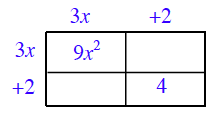### Home > PC > Chapter 1 > Lesson 1.1.2 > Problem1-25

1-25.

Explain why $\left(3x + 2\right)^{2} ≠ 9x^{2} + 4$? Find the correct expansion.

Complete the generic rectangle below and simplify. What is missing?The equation will be true if $x$ is a certain value. What is that value?